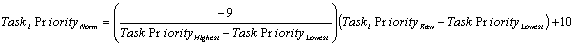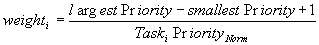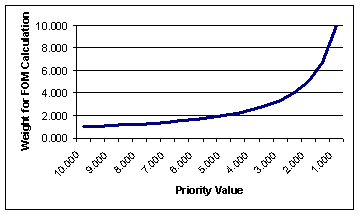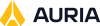The Figure of Merit calculation uses the task priorities to help determine which of the possible deconflicted schedule solutions is most preferred. STK Scheduler allows you to determine the priority scale range and the importance convention of tasks and resources independently. Task priority range and convention are defined on the Task Priority Tab of the Schedule Properties Form. You are then free to define task priorities for each task using the General Tab of the Task Definition form.Priorities are then converted to weight to be used in the FOM calculation using the function below. The weight ranges from 1 to 10 with 10 being the highest.This gives the following relations between the normalized priority value and the weight used in the FOM calculation:The weight value is then passed to the FOM calculation.